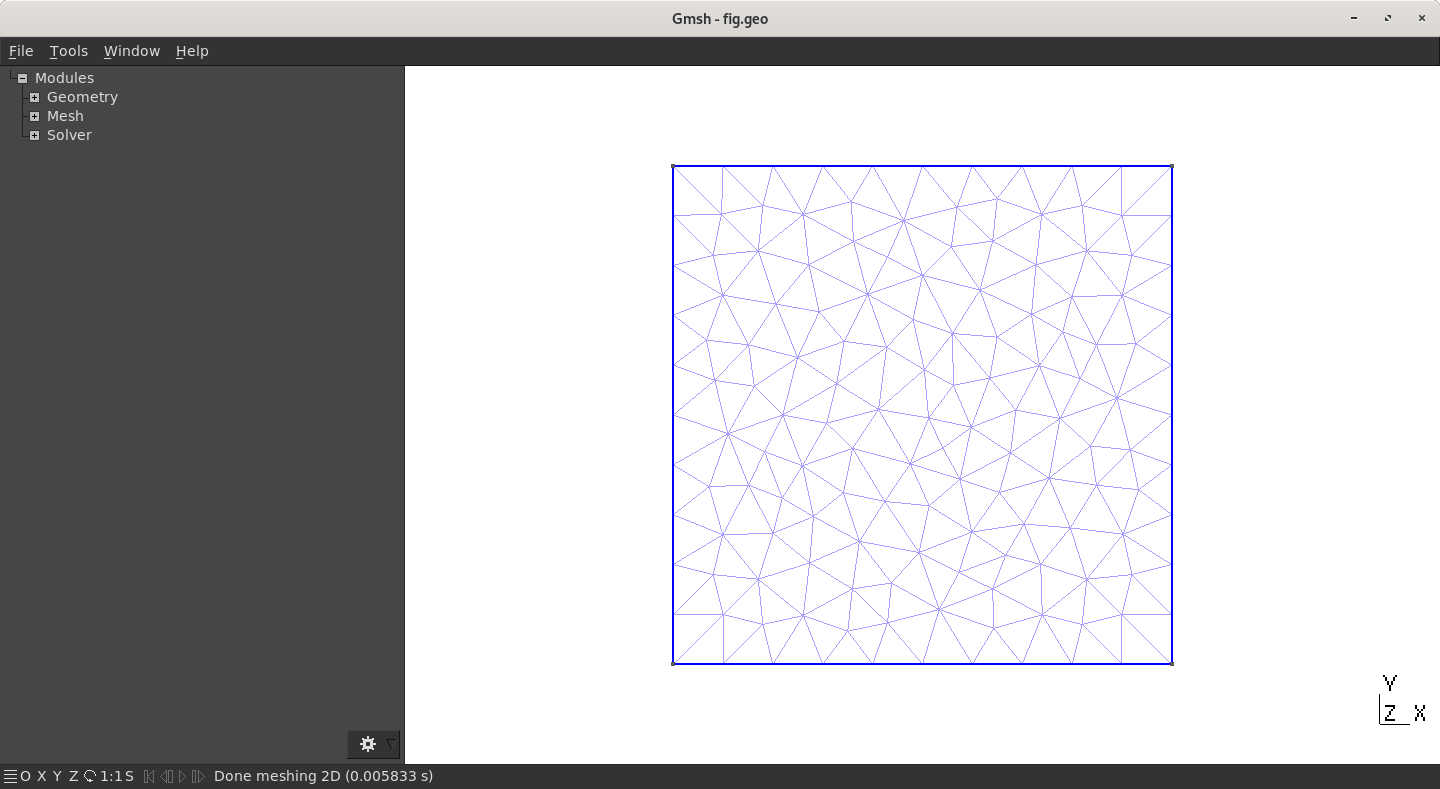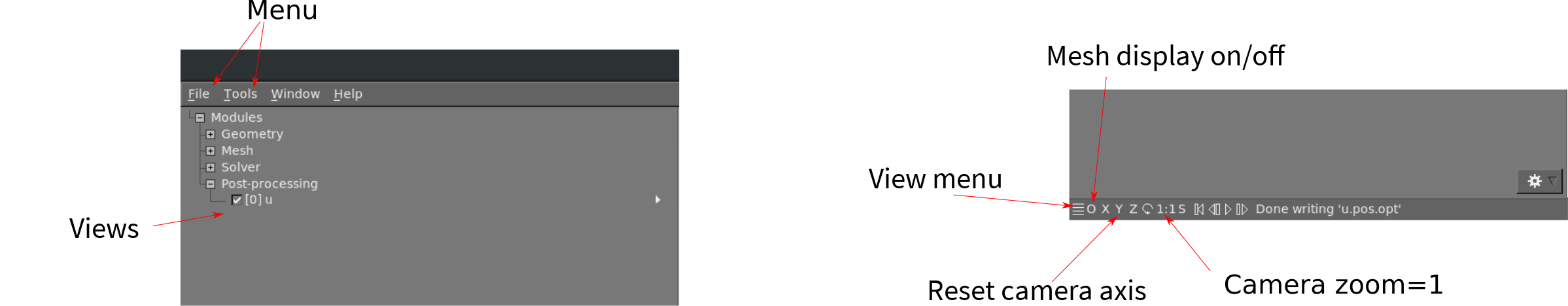# First Mesh

## My first square

### Let’s go

1. Copy/paste the following code in a new file, named e.g. `square.geo`:

`````` h = 1;                     // Characteristic length of a mesh element
Point(1) = {0, 0, 0, h};   // Point construction
Point(2) = {10, 0, 0, h};
Point(3) = {10, 10, 0, h};
Point(4) = {0, 10, 0, h};
Line(1) = {1,2};            //Lines
Line(2) = {2,3};
Line(3) = {3,4};
Line(4) = {4,1};
Curve Loop(1) = {1,2,3,4};   // A Boundary
Plane Surface(1) = {1};     // A Surface
Physical Surface(1) = {1};  // Setting a label to the Surface
``````
2. In GMSH, go to `files->open` (`CTRL+o`) and open the file, or type `gmsh square.geo` in a terminal (warning: this open a new instance of GMSH (which is very light by the way!)).

3. A square should have appear in GMSH’s windows. The camera can be adjusted using the mouse: rotating (`left click`), translating (`right click`) or zooming (`wheel`). At bottom left of GMSH’s windows, camera can be reseted using `X,Y,Z` and `1:1` (scale) buttons.

4. The square can now be meshed by typing `2` on the keyboard (or maybe `shift` + `2`) or using the menu: `Mesh->2D`Mesh of a squareMenu and Camera

### Log

GMSH is provided with a log console that can be displayed by clicking on the bottom banner of the window. For the square example, you should have something like

``````Info    : Reading 'square.geo'...
Info    : Done reading 'square.geo'
Info    : Finalized high order topology of periodic connections
Info    : Meshing 1D...
Info    : Meshing curve 1 (Line)
Info    : Meshing curve 2 (Line)
Info    : Meshing curve 3 (Line)
Info    : Meshing curve 4 (Line)
Info    : Done meshing 1D (0.000981 s)
Info    : Meshing 2D...
Info    : Meshing surface 1 (Plane, Delaunay)
Info    : Done meshing 2D (0.017519 s)
Info    : 142 vertices 286 elements
``````

This shows that GMSH starts by meshing the four lines (`Meshing 1D...`) then the surface. In this example, 142 vertices have been created for a total of 286 elements (lines and triangles).

Mesh can be saved using `CTRL + Shift + s` or using graphical interface (`file->save mesh`). By default, the extension of the mesh is `.msh` and of the same root as the `.geo` file (i.e. `square.geo` leads to `square.msh`).

## Code analyse

### Parameter `h`

On the first line of our code is defined a variable, `h`, which is the characteristic length of the elements. This size is precised at each `Point` elementary entity.

Let us play with it (check the tips bellow first):

1. Modify the parameter `h`, remesh and observe the result
2. Anisotropic mesh: change only the mesh size of `Point(2)`, for example with `h/10`. Remesh and observe the result
When the file has been modified, there is no need to relaunch GMSH: simply press `0` (zero) (or maybe `shift` + `0`). This forces GMSH to reset and re-read the current file.

### `Point` entity

In GMSH, a volume (3D) is defined by its surfaces, a surface (2D) by its (closed) curves and a curve (1D) by its endpoints. Each of these, `Volume`, `Surface`, `Curve` and `Point` are named elementary entities in GMSH. The first one that is studied here are the 0D entity: `Point`. They are defined as follows

``````Point(index) = {x, y, z, h};
``````

where the parameters are:

• `index`: unique identifier (`int` > 0) of the `Point`. This number has to be set by the user, either manually or using `newp` which returns the next available index for `Point` (`newp` = NEW Point).
• `x`, `y`, `z`: cartesian coordinate of the `Point`
• `h`: mesh element size around the `Point`

Apply the following change to `square.geo`:

1. Move some `Point` to obtain a rectangle (instead of a square)
2. Move some `Point` to get a quadrangle not rectangular at all

### `Line` and `Curve Loop` entities

#### `Line`

It is an oriented segment linking two `Point`:

``````  Line(index) = {PointA, PointB};
``````

Avec comme paramètres :

• `index`: unique identifier (`int` > 0) of the `Line`. As for `Point`,this number has to be set by the user, either manually or using `newl` (next available index for `Line`).
• `PointA`: index of the starting `Point`
• `PointB`: index of the ending `Point`
A `Line` is oriented!

#### `Curve Loop`

Closed loop of `Curve` (`Line` being a `Curve`) that represent a boundary (for a `Surface` generally):

``````Curve Loop(index) = {Curve1, Curve2, ..., CurveN};
``````

Where:

• `index`: Unique identifier (`int` > 0) of the `Curve Loop` (`newll` can be used)
• `CurveX`: index of the Xth `Curve` (or `Line`). If this quantity is negative (e.g. -10) then the `Curve` is oriented backwardly.

Let’s do a “L-shape” geometry in a new file `L.geo` as proposed in the below figure (Set a mesh refinement to `h`=1). You can copy/paste the previous `square.geo` file to help yourself.L Shape geometry (with h=1 !)

### `Surface` entity

A `Surface` is defined by its boundaries: their `Curve Loop` (possibly more than one):

``````Plane Surface(index) = {CurveLoop1, CurveLoop2, ..., CurveLoopN};
``````
• `index`: Unique identifier (`int` > 0) of the `Surface` (`news` can be used)
• `CurveLoopX`: index of the Xth `Curve Loop`. As for the `Line`, this quantity can be negative (e.g. -10) to backwardly orient a `Curve Loop`.

Modify the “L-shape” geometry to add a hole inside as on the below figure. You will have to add `Point` and `Line` obvsiouly, but also a new `Curved Loop`. The `Surface` must then be defined by the 2 loops!L-Shape avec un trou carré

### `Elementary Entities` vs. `Physical Entities`

The last line of the file`square.geo` is:

``````Physical Surface(1)={1};
``````

This is particular to GMSH and do not affect the geometry rather than the output mesh file. The command is the same for `Volume`, `Surface`, `Curve` and `Point`:

``````Physical Volume/Surface/Line/Point(index) = {entity1, entity2, ..., entityN};
``````

Basically, GMSH can set a unique identifier (`index`) to a set of entities (to regroup them). This new identifier has nothing to do with the previous one you have defined. The output file do not contain the `elementary` index but only the `physical` ones. Moreover, only the entities that have a `physical` tag are saved to the disk! This is explained in detail later.